集合框架系列教材 （十二）- 关系与区别 - Java ArrayList和HashSet的区别 集合框架系列教材 （十四）- 关系与区别 - HashMap和Hashtable之间的区别5分40秒

ArrayList 插入，删除数据慢
ArrayList是顺序结构，所以定位很快，指哪找哪。 就像电影院位置一样，有了电影票，一下就找到位置了。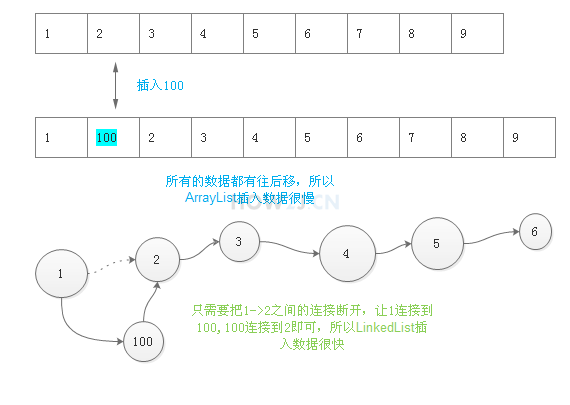## 插入数据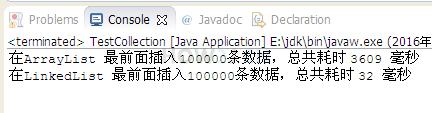package collection; import java.util.ArrayList; import java.util.LinkedList; import java.util.List; public class TestCollection { public static void main(String[] args) { List<Integer> l; l = new ArrayList<>(); insertFirst(l, "ArrayList"); l = new LinkedList<>(); insertFirst(l, "LinkedList"); } private static void insertFirst(List<Integer> l, String type) { int total = 1000 * 100; final int number = 5; long start = System.currentTimeMillis(); for (int i = 0; i < total; i++) { l.add(0, number); } long end = System.currentTimeMillis(); System.out.printf("在%s 最前面插入%d条数据，总共耗时 %d 毫秒 %n", type, total, end - start); } }

## 定位数据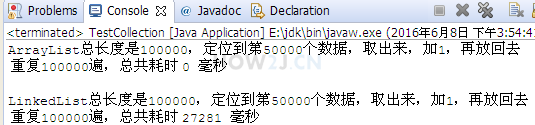package collection; import java.util.ArrayList; import java.util.LinkedList; import java.util.List; public class TestCollection { public static void main(String[] args) { List<Integer> l; l = new ArrayList<>(); modify(l, "ArrayList"); l = new LinkedList<>(); modify(l, "LinkedList"); } private static void modify(List<Integer> l, String type) { int total = 100 * 1000; int index = total/2; final int number = 5; //初始化 for (int i = 0; i < total; i++) { l.add(number); } long start = System.currentTimeMillis(); for (int i = 0; i < total; i++) { int n = l.get(index); n++; l.set(index, n); } long end = System.currentTimeMillis(); System.out.printf("%s总长度是%d，定位到第%d个数据，取出来，加1，再放回去%n 重复%d遍，总共耗时 %d 毫秒 %n", type,total, index,total, end - start); System.out.println(); } }

## 练习-在后面插入数据

姿势不对,事倍功半! 点击查看做练习的正确姿势

## 答案-在后面插入数据1分58秒 本视频采用html5方式播放，如无法正常播放，请将浏览器升级至最新版本，推荐火狐，chrome，360浏览器。 如果装有迅雷，播放视频呈现直接下载状态，请调整 迅雷系统设置-基本设置-启动-监视全部浏览器 (去掉这个选项)。 chrome 的 视频下载插件会影响播放，如 IDM 等，请关闭或者切换其他浏览器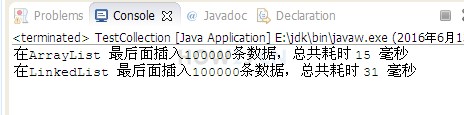package collection; import java.util.ArrayList; import java.util.LinkedList; import java.util.List; public class TestCollection { public static void main(String[] args) { List<Integer> l; l = new ArrayList<>(); insertFirst(l, "ArrayList"); l = new LinkedList<>(); insertFirst(l, "LinkedList"); } private static void insertFirst(List<Integer> l, String type) { int total = 1000 * 100; final int number = 5; long start = System.currentTimeMillis(); for (int i = 0; i < total; i++) { //直接add就表示插入在最后 l.add(number); } long end = System.currentTimeMillis(); System.out.printf("在%s 最后面插入%d条数据，总共耗时 %d 毫秒 %n", type, total, end - start); } }

## 练习-在中间插入数据

姿势不对,事倍功半! 点击查看做练习的正确姿势

## 答案-在中间插入数据2分4秒 本视频采用html5方式播放，如无法正常播放，请将浏览器升级至最新版本，推荐火狐，chrome，360浏览器。 如果装有迅雷，播放视频呈现直接下载状态，请调整 迅雷系统设置-基本设置-启动-监视全部浏览器 (去掉这个选项)。 chrome 的 视频下载插件会影响播放，如 IDM 等，请关闭或者切换其他浏览器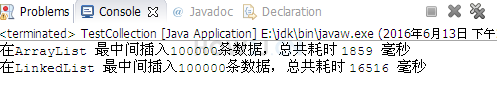package collection; import java.util.ArrayList; import java.util.LinkedList; import java.util.List; public class TestCollection { public static void main(String[] args) { List<Integer> l; l = new ArrayList<>(); insertFirst(l, "ArrayList"); l = new LinkedList<>(); insertFirst(l, "LinkedList"); } private static void insertFirst(List<Integer> l, String type) { int total = 1000 * 100; final int number = 5; long start = System.currentTimeMillis(); for (int i = 0; i < total; i++) { //把当前容器的一半的位置，插入数据 l.add(l.size()/2,number); } long end = System.currentTimeMillis(); System.out.printf("在%s 最中间插入%d条数据，总共耗时 %d 毫秒 %n", type, total, end - start); } }

 集合框架系列教材 （十二）- 关系与区别 - Java ArrayList和HashSet的区别 集合框架系列教材 （十四）- 关系与区别 - HashMap和Hashtable之间的区别

HOW2J公众号，关注后实时获知最新的教程和优惠活动，谢谢。2021-07-30 最后添加

2 个答案

15830338618aa

Java小菜鸡Rookie

2021-07-05 练习 - 插入数据两问
 莫諾 1.比较ArrayList和LinkedList在最后面插入100000条数据，谁快谁慢？为什么？ 2.在List的中间位置，插入数据，比较ArrayList快，还是LinkedList快，并解释为什么？ 结论一: LinkedList快一点；因为最后面插入数据时ArrayList要后移一位，而LinkedList直 接把数据链接到最后一位元素上，中间也没有断链的动作； 结论二: ArraList快；因为在中间插入数据时ArrayList定位到中间位置非常快，而LinkedList在 中间插入数据时，每次都要一个接一个定位到中间的位置，然后才有插入数据的动作； ```//练习在后面插入数据 private static void insertLast(List l, String type) { int t = 100000; final int number = 5; long start = System.currentTimeMillis(); for (int i = 0; i < t; i++) { l.add(i,number); } long end = System.currentTimeMillis(); System.out.printf("在%s最后面插入%d条数据，总共耗时%d毫秒%n", type, t, end - start); } public static void main(String[] args) { List l; l = new ArrayList<>(); insertLast(l, "ArraList"); l = new LinkedList<>(); insertLast(l, "LinkedList"); } // 练习在中间插入数据 private static void insertMiddle(List l, String type) { int t = 100000; final int number = 5; long start = System.currentTimeMillis(); for (int i = 0; i < t; i++) { int index = i/2; l.add(index,number); } long end = System.currentTimeMillis(); System.out.printf("在%s中间插入%d条数据，总共耗时%d毫秒%n", type, t, end - start); } public static void main(String[] args) { List l; l = new ArrayList<>(); insertMiddle(l, "ArraList"); l = new LinkedList<>(); insertMiddle(l, "LinkedList"); }```

2020-07-07 两提
2020-06-12